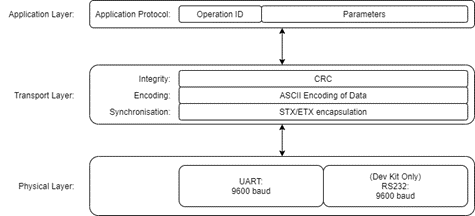# Astronode Serial Commands

## Introduction​

This section describes how to use and interact with the Astronode S module. It is intended for users and integrators of Astrocast products. It provides specifications for the communication protocol over UART and various IO lines.

## What hardware products does this cover?​

This covers all Astrocast Products that include an Astronode™ S module:

## Conventions and Data Format​

Hexadecimal values are always prefixed by the two characters 0x. e.g. 0x8000 is equal to the decimal value 32768.

### Bit Numbering Convention​

The first bit in a field to be transmitted (i.e. the right-most justified bit when writing a byte) is defined to be "Bit 0"; the following bit is called "Bit 1" and so on up to "Bit N-1". Bit 0 is the Least Significant bit (LSb). Bit N-1 is the Most Signficant bit (MSb). In this documentation bit fields are written as X.Y where X is the byte position and Y is the bit position. E.g. 5.0 is byte 5, bit 0 (LSb of byte field); 6.1 is byte 6, bit 1; 8.7 is byte 8, bit 7 (MSb of byte field).

Bit N-1                       Bit0
N-Bit Data Field
MSbLSb

### Byte Ordering​

Little-endian format shall be used. When a field expresses a value larger than a single byte the Least Significant Byte (LSB) shall be the first transmitted byte, and the Most Significant Byte (MSB) shall be transmitted last.

Transmission of the 4 byte number 0x12345678 will result in the follow byte transmission order:

Byte 0 (LSB)Byte 1Byte 2Byte 3 (MSB)
0x780x560x340x12

### Type Names​

A byte is 8-bits in length. UInt8 is an unsigned byte. Int8 is a signed byte.

A nibble is half a byte, 4 bits in length. A byte, UInt8, or Int8 is composed of 2 nibbles: high and low. E.g. 0x78: 7 is the high nibble and 8 is the low nibble.

A short word is 16-bits in length (i.e. 2 bytes). UInt16 is an unsigned short. Int16 is a signed short.

A word is 32-bits in length (i.e. 4 bytes). UInt32 is an unsigned word. Int32 is a signed word.

A long word is 64-bits in length (i.e. 8 bytes). UInt64 is an unsigned long. Int64 is a signed long.

Numbering of bytes in a field is done from LSB as byte 0 to MSB as byte N-1 in an N-byte field.

Byte N-1Byte N-2etc...Byte 1Byte 0
N-byte Data Field
MSBLSB

## Protocol Layering Overview​

The protocol is described in 3 layers:

1. Physical layer: uart and optionally RS232.
2. Transport layer: message synchronisation, encoding and integrity checking.
3. Application layer: messages of operation IDs and parameters